1　 2　3　 4　 5　 6　 7　 8　 9　 10　 11　 12　 13　 14 　15

A　S　O　R　 T　 I　 N　G　 E　 X 　A　　M　 P　　L　　E

A　A　E　E　 T　 I　 N　G　 O　 X 　S　　M　 P　　L　　R

A　A　E

A　A

A

L　I　 N　G　 O　P　 M　　R　 X　　T　　S

L　I　 G　M　 O　P　 N

G　I　 L

I　 L

I

N　P　O

O　P

P

S 　　T　　X

T　　X

T

A　A　E　E　 G　 I　 L　M　N　O　P　 R　　S　　T　　X

CN =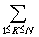(N+1+CK-1+CN-K)　　　　　　　　 C0=C1=0

=N+1+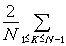CK

CN-1 = N +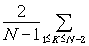CK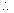CN =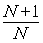CN-1 + 2

:

:

CN =(N+1)(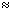2NlgN